# RShiny for prototyping + plotting two different user-input variables

4 minute read

Last week after some team meetings I started using RShiny to prototype a webapp for a project I’m working on to show users how many resources (i.e. time, memory, CPUs) they might need for running various bioinformatics programs on our computing cluster. This was after several projects we had were stalled out on the public-facing piece due to our single front-end developer leaving and the rest of our collective lack of experience. There was one app, RefChef, built with React/Ember, and a few others had been started in Vue/Nuxt. None of us had done anything in Javascript (I had done a little d3 before but it was more of the copy d3 examples and adjust values type) and certainly not any JS frameworks. The learning curve turned out to be pretty big given that software engineering tends to be our last priority and research/analyses our first priority - several months later I still haven’t quite been able to combine existing components into a working interactive page.

Pivot to RShiny. I have so much more experience and familiarity with R, including writing R packages. The turnaround time to a working prototype has been drastically less. I was able to get a basic app up of what I wanted in about an hour and finish the whole thing in another hour or 2 including reading through tutorials.

Getting started was a matter of starting an RShiny project from RStudio, once you make it they immediately start you up with an `app.R` example you can build off of.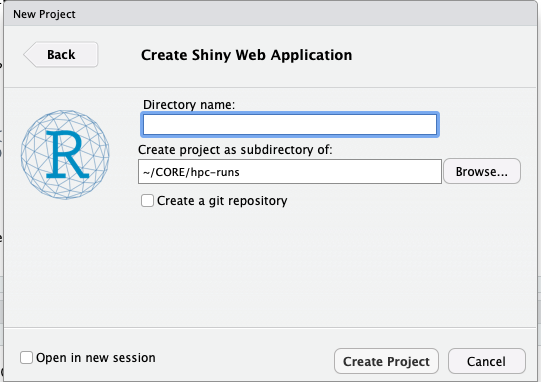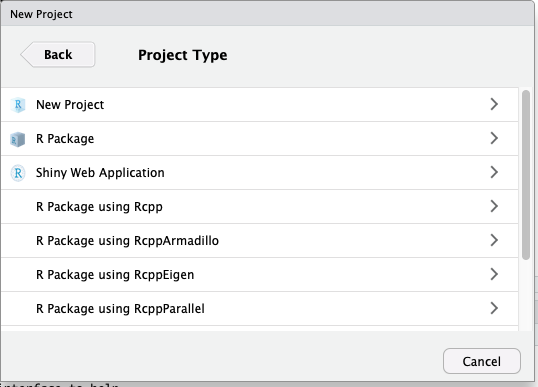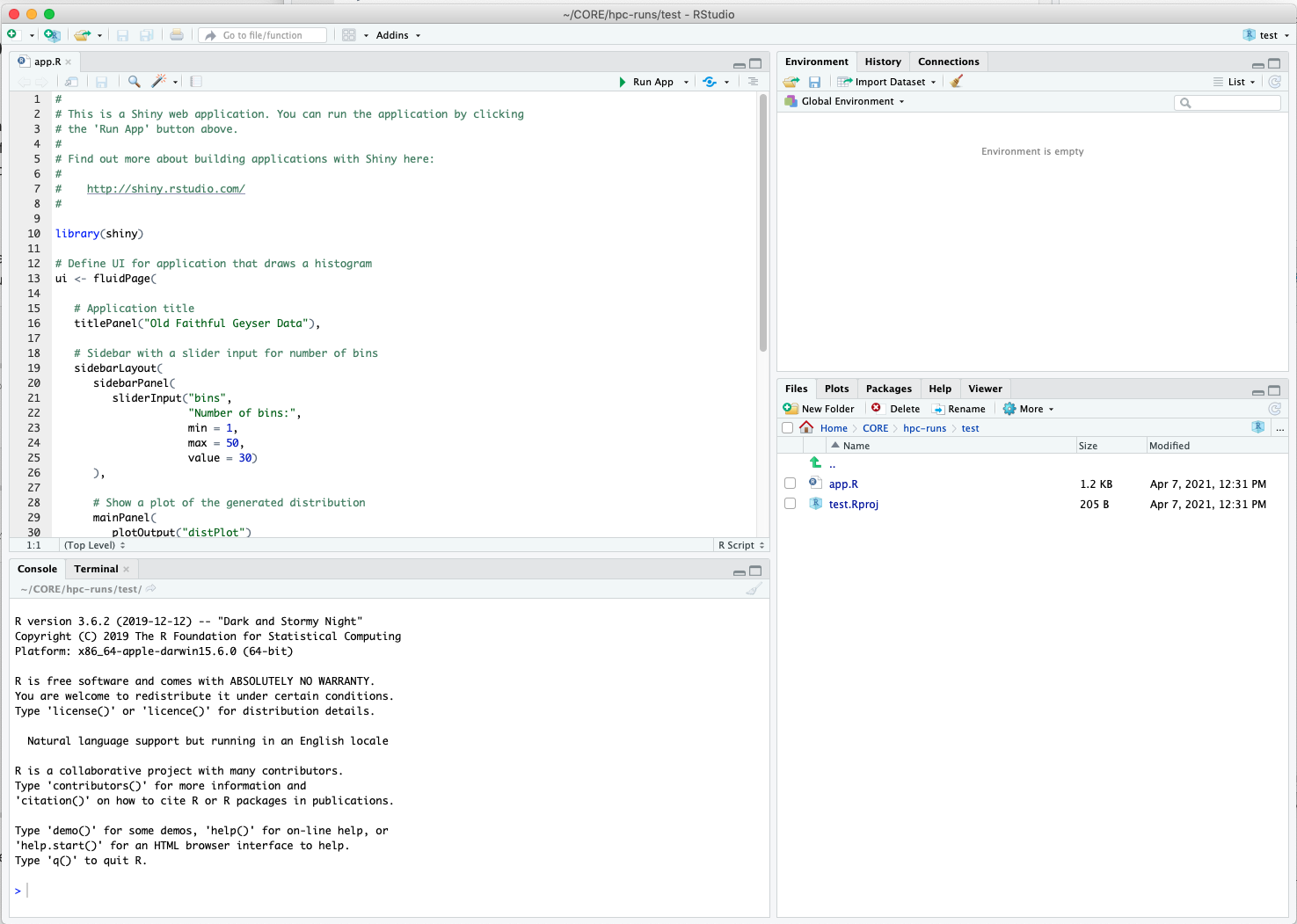Snapshots of the Project Type menu, the new Shiny project menu, and what it looks like in RStudio right after.

In terms of resources for learning RShiny, I’ve just been following along with the tutorials posted from their page on RStudio. They have a wide mass of videos and articles. What was most useful for me were the basics, understanding reactive components, and the Database basics. The next step would be to switch to using `pool` and `dplyr` to query an actual DB rather than feed in a CSV, make the plot interactive / into a dashboard, and be able to plot multiple variables categorized by color on a single plot. These are all extras though, I already have my MVP.

You can see the prototype on aguang.shinyapps.io. With this I can now go back to focusing on the backend, i.e. pulling more data, standardizing units, setting up a DB.

# Plotting two different user-input variables

Of course some things I had to figure out on my own. One was that I wanted the user to be able to choose the variables they plotted on a scatter plot and also have nice axis labels. At first I thought I could just pass in `input\$var1` and `input\$var2` to `ggplot`, but the problem is that they would get interpreted as the actual strings rather than variable names (a common difficulty in other areas with `tidyverse`… I have memories of dealing with this in other instances but now can’t remember how) This would result in a plot of a single point that was string1 vs string2.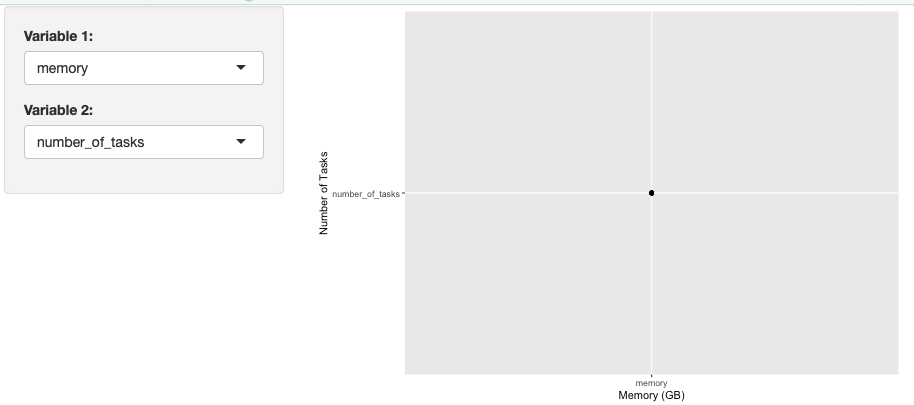Selecting memory and number_of_tasks causes the actual strings to be plotted rather than the data in the columns with those names.

The correct way to do did not seem to be found in the RShiny tutorials or a quick StackOverflow search.

The solution I came up with was to make use of `dplyr::rename`, a new function I learned about. Basically, I wrote three reactive functions. One renames the variables of interest to `var1` and `var2` so that when I go to `ggplot` I can use a single line that passes in `var1` and `var2` as column names instead of strings. The other two are just `switch` statements that take in the input and output strings for axis labels, they are then run inside `xlab` and `ylab` respectively.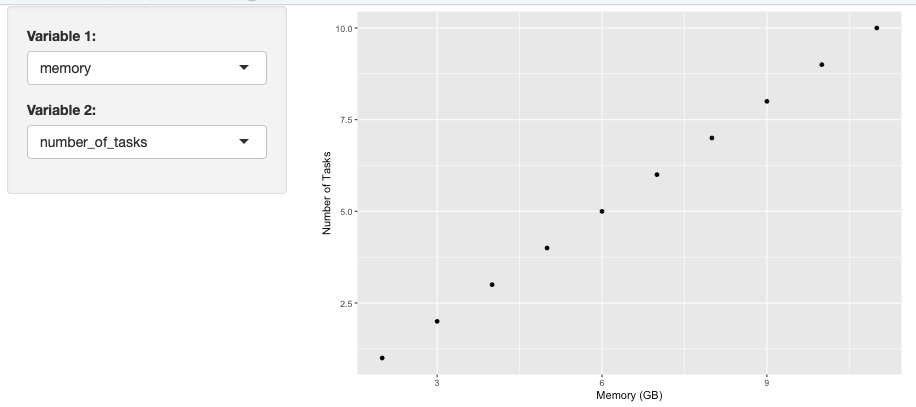Selecting memory and number_of_tasks now plots the data from the columns with those names.

The code to reproduce is below.

``````
library(shiny)
library(tidyverse)

ui <- fluidPage(
sidebarPanel(
selectInput(inputId = "var1",
label = "Variable 1:",
choices = c("number_of_tasks","cpus_per_task","memory","time","file_sizes"),
selected = "memory"),

selectInput(inputId = "var2",
label = "Variable 2:",
choices = c("number_of_tasks","cpus_per_task","memory","time","file_sizes"),
selected = "file_sizes")
),
mainPanel(
plotOutput("distPlot"))
)

server <- function(input, output) {
# this is example data for a minimal example
data <- data.frame(number_of_tasks=seq(1,10,1),
cpus_per_task=seq(1,10,1),
memory=seq(2,11,1),
time=seq(1,10,1),
file_sizes=seq(1,10,1))

# renames variables of interest to var1 and var2 for ggplot
plotInput <- reactive({
rename(data, var1=input\$var1, var2=input\$var2)
})

# reactive component for desired labels
var1_lab <- reactive({
switch(input\$var1,
"number_of_tasks" = "Number of Tasks",
"cpus_per_task" = "CPUs per Task",
"memory" = "Memory (GB)",
"time" = "Time (s)",
"file_sizes" = "Input Size (GB)")
})

var2_lab <- reactive({
switch(input\$var2,
"number_of_tasks" = "Number of Tasks",
"cpus_per_task" = "CPUs per Task",
"memory" = "Memory (GB)",
"time" = "Time (s)",
"file_sizes" = "Input Size (GB)")
})

output\$distPlot <- renderPlot(ggplot(plotInput(), aes(x=var1,y=var2)) + geom_point() +
xlab(var1_lab()) +
ylab(var2_lab()))
}

shinyApp(ui = ui, server = server)
``````

Tags:

Categories:

Updated: